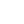# Algorithms: Insertion Sort with Javascript

By Henri ParviainenInsertion sort is one of the basic sorting algorithms that is always taught in every algorithms and data structures course. Every software engineer should have a solid understanding of insertion sort. In this quick tutorial, you will learn how to implement insertion sort algorithm in Javascript.

## How insertion sort works

Insertion sort works by dividing the input array into two sub-arrays: sorted array and unsorted array. Then, the algorithm iterates through the elements in the unsorted part and places values in the correct position in the sorted part. This will eventually sort the whole array.## Example

In the following code example, you can see how to sort an unsorted array using insertion sort with Javascript.

``````const insertionSort = (arr) => {
var currentVal;

for (var i = 1; i < arr.length; i++) {
currentVal = arr[i];
for (var j = i - 1; j >= 0 && arr[j] > currentVal; j--) {
arr[j + 1] = arr[j];
}
arr[j + 1] = currentVal;
}

return arr;
};

insertionSort([2, 11, 4, 36, 15]);

// [2, 4, 11, 15, 36]``````

## Use cases and complexity

In the worst case, the time complexity for insertion sort is `O(n2)`.

Insertion sort excels when input data is already nearly sorted.

In conclusion, insertion sort is quite a decent general-purpose sorting algorithm.

You might also be interested in checking my posts on selection sort and bubble sort.